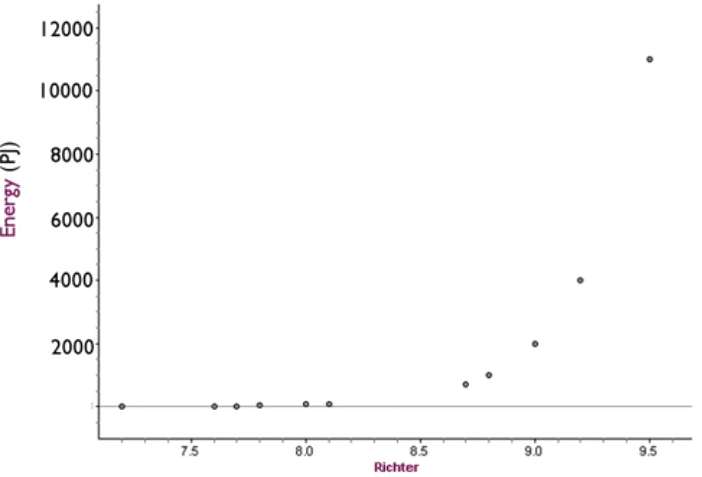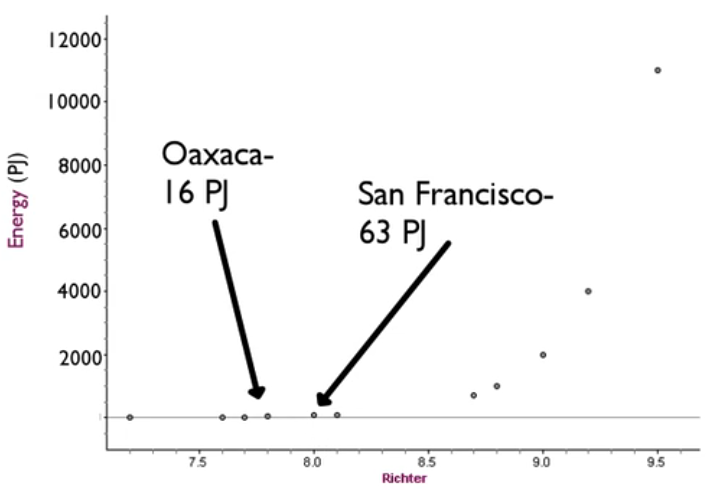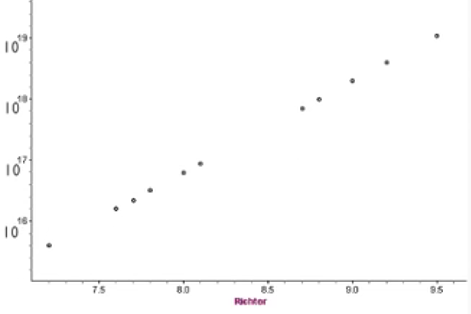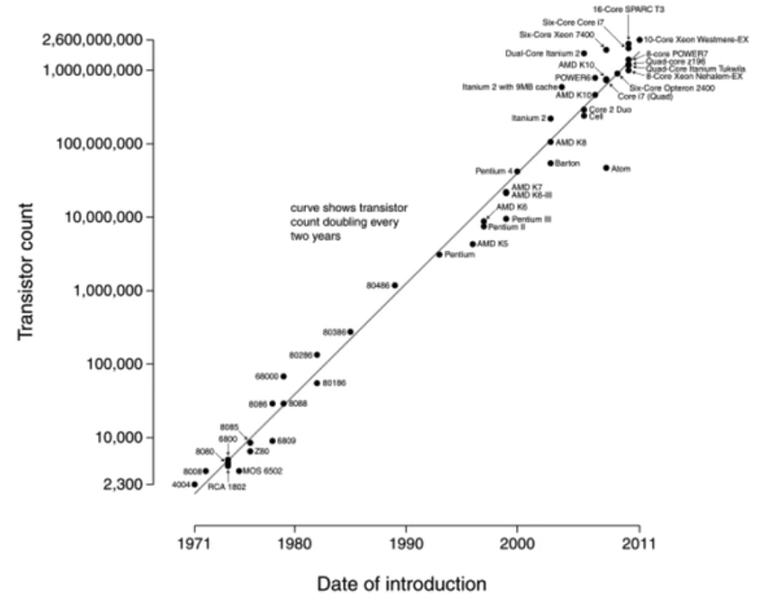+

# Logarithmic Scales

Author: Sophia Tutorial
##### Description:

This lesson will explain logarithmic scales.

(more)

Sophia’s self-paced online courses are a great way to save time and money as you earn credits eligible for transfer to many different colleges and universities.*

No credit card required

37 Sophia partners guarantee credit transfer.

299 Institutions have accepted or given pre-approval for credit transfer.

* The American Council on Education's College Credit Recommendation Service (ACE Credit®) has evaluated and recommended college credit for 33 of Sophia’s online courses. Many different colleges and universities consider ACE CREDIT recommendations in determining the applicability to their course and degree programs.

Tutorial
what's covered
Logarithmic scales are used to adjust the axes on a plot to make it easier to interpret. This tutorial will discuss:
1. Logarithmic Scales
2. Moore's Law

## 1. Logarithmic Scales

The best way to explain logarithmic scales is to show you how it works. Here's a list of earthquakes based on where and when they occurred.

Location Country Year Energy (in PJ) Richter
Lisbon Portugal 1755 2000 9
San Francisco USA 1906 63 8
Hawke's Bay New Zealand 1931 32 7.8
Valdivia Chile 1960 11,000 9.5
Anchorage USA 1964 4,000 9.2
Tangshan China 1976 32 7.8
Vrancea Romania 1977 4 7.2
Mexico City Mexico 1985 89 8.1
Jiji Taiwan 1999 16 7.6
Indian Ocean Indonesia 2004 4,000 9.2
Sumatra Indonesia 2005 710 8.7
Sichuan China 2008 63 8
Sumatra Indonesia 2010 22 7.7
Pelluhue Chile 2010 1,000 8.8
Tohoku Japan 2011 2,000 9
Oaxaca Mexico 2012 16 7.6

More importantly, the focus on this table is the energy released in petajoules, which is millions of millions of joules and their values on the Richter scale. You can see that in 2004, Christmas Day, a tsunami struck Indonesia. You can also see the famous San Francisco earthquake of 1906.

Look at the data on a scatter plot. The data actually follows a curve. As the Richter value goes up, so does the energy released from the earthquake. But it's a curve, it's not a straight line.Here is the problem: Oaxaca earthquake released 16 petajoules of energy, which is a lot. However, the San Francisco earthquake released almost four times as much energy, yet it looks very similar to the Oaxaca earthquake on this particular scale.It's difficult to interpret the differences even though the difference is large. But it's hard to tell because the values differ by such a large order of magnitude.

To see those differences, it's to use a logarithmic scale instead of beneficial, instead of a standard scale. A standard scale uses equal increments.

term to know
Logarithmic Scale
A scale that uses equal measurements to represent equal ratios (usually multiplying by 10) rather than equal intervals.

A logarithmic scale will use equal ratios, so each distance represents 1,000, no matter how many increments used. So it might be 10, and then times 10 again, and times 10 again, etc. So you get to 10,000. As opposed to 10 being way way, way down here near the x-axis, it's actually up here. So you can see those differences more clearly.

Compare the standard scale for earthquake data vs. a logarithmic scale. This is energy in 10 to the 16th power, 10 to the 17th power, etc. Data that appear exponentially curved on a standard scale will often end up appearing linear on a logarithmic scale. This is an important piece to consider.## 2. Moore's Law

One big example of a logarithmic scale being useful is something called Moore's Law. This law focused on a trend involving components on microcompressors on a circuit. Gordon Morre said that the number of components in circuits has doubled every year since the invention of the circuit, and the trend would continue for at least 10 years. So, why does this matter?

Well, if you look at what actually ended up happening, it actually continued much, much further into the future than he had predicted. It does appear that between every one and two years, typically around every year, the number of microprocessor transistors had in fact doubled. This is viewed on a logarithmic scale and it looks linear. Doubling every year is actually an exponential growth curve.term to know
Moore's Law
A trend in technology for the number of components on microprocessors to double every year. It was first posited by Gordon E. Moore in 1965, and has more or less continued to the present day.

But here, it looks more linear. So examples of employing a logarithmic scale to show points lying on a line instead of an exponential curve are examples like the Richter scale, examples like Moore's law, but also examples like the decibel scale, which measures sound, and the pH scale, which measures acidity.

did you know
Moore's Law is named after the co-founder of the company Intel, Gordon E. Moore.

summary
Logarithmic scales are used to show data points that are very far apart. Typically, a rule of thumb is by factors of 100 or more. So if something was 1,000 versus a value of 10, you might want to use a logarithmic scale. Often, data points that show an exponential curve on a standard scale will form a line on a logarithmic scale. And so, we saw that with both the earthquake data and the Moore's Law-- the graph that we showed. So, we talked about logarithmic scales and used Moore's law as an example

Source: This work is adapted from Sophia author Jonathan Osters.

Terms to Know
Logarithmic Scale

A scale that uses equal measurements to represent equal ratios (usually multiplying by 10) rather than equal intervals.

Moore's Law

A trend in technology for the number of components on microprocessors to double every year. It was first posited by Gordon E. Moore in 1965, and has more or less continued to the present day.

Rating